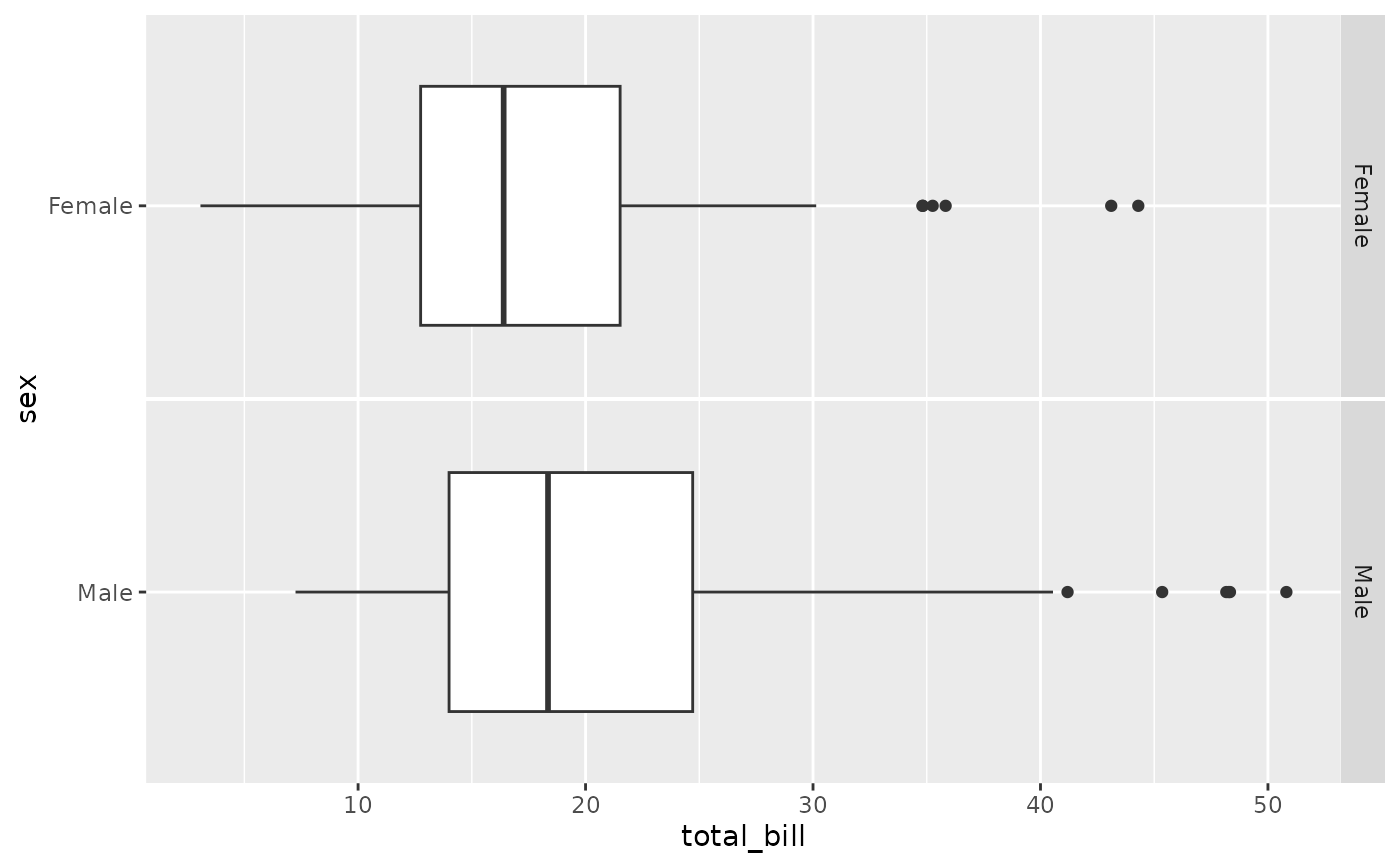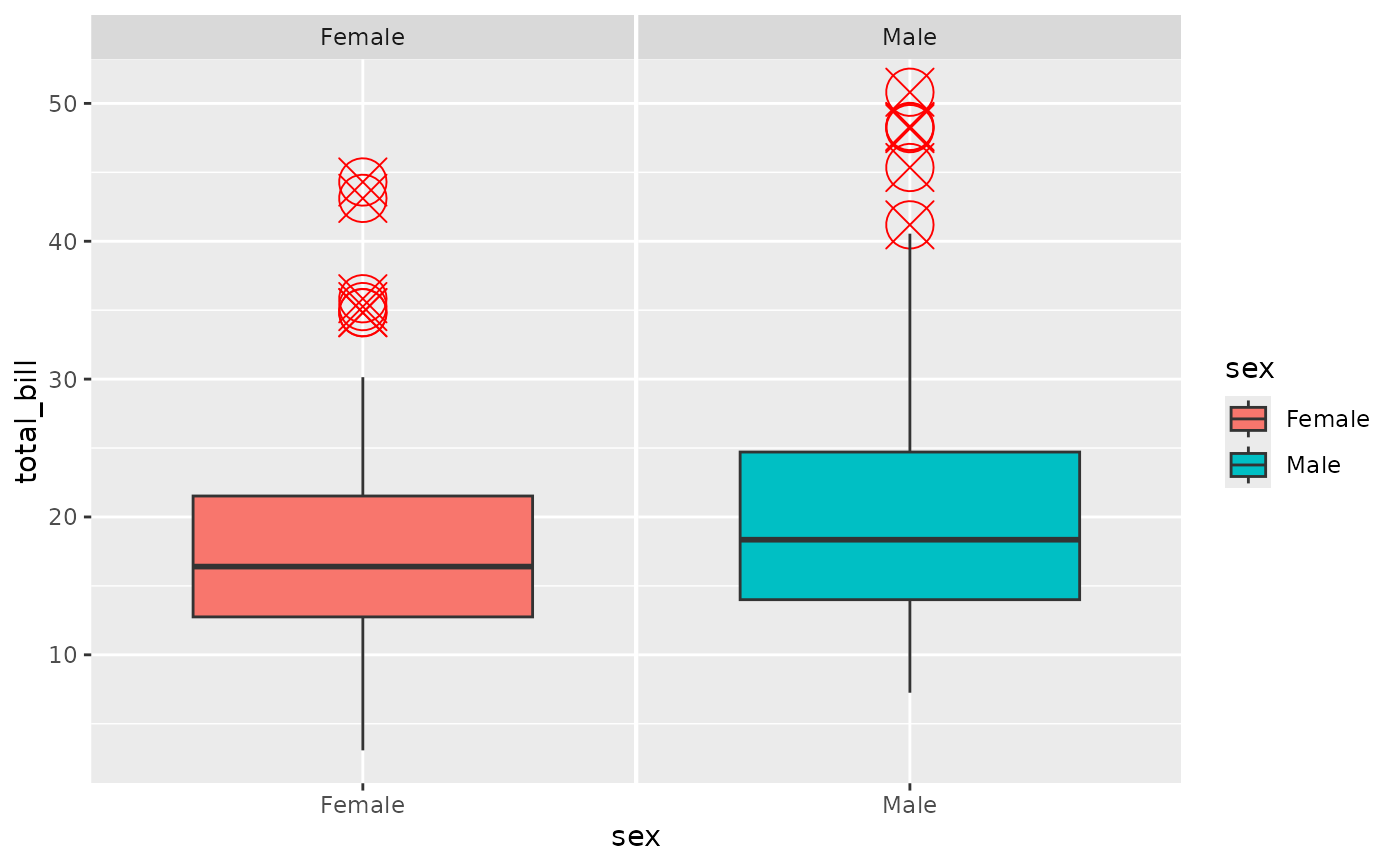Make a box plot with a given data set. ggally_box_no_facet will be a single panel plot, while ggally_box will be a faceted plot

ggally_box(data, mapping, ...)

ggally_box_no_facet(data, mapping, ...)

## Arguments

data data set using aesthetics being used other arguments being supplied to geom_boxplot

Barret Schloerke

## Examples

# Small function to display plots only if it's interactive
p_ <- GGally::print_if_interactive

data(tips, package = "reshape")
p_(ggally_box(tips, mapping = ggplot2::aes(x = total_bill, y = sex)))p_(ggally_box(tips, mapping = ggplot2::aes_string(x = "total_bill", y = "sex")))p_(ggally_box(
tips,
mapping        = ggplot2::aes_string(y = "total_bill", x = "sex", color = "sex"),
outlier.colour = "red",
outlier.shape  = 13,
outlier.size   = 8
))